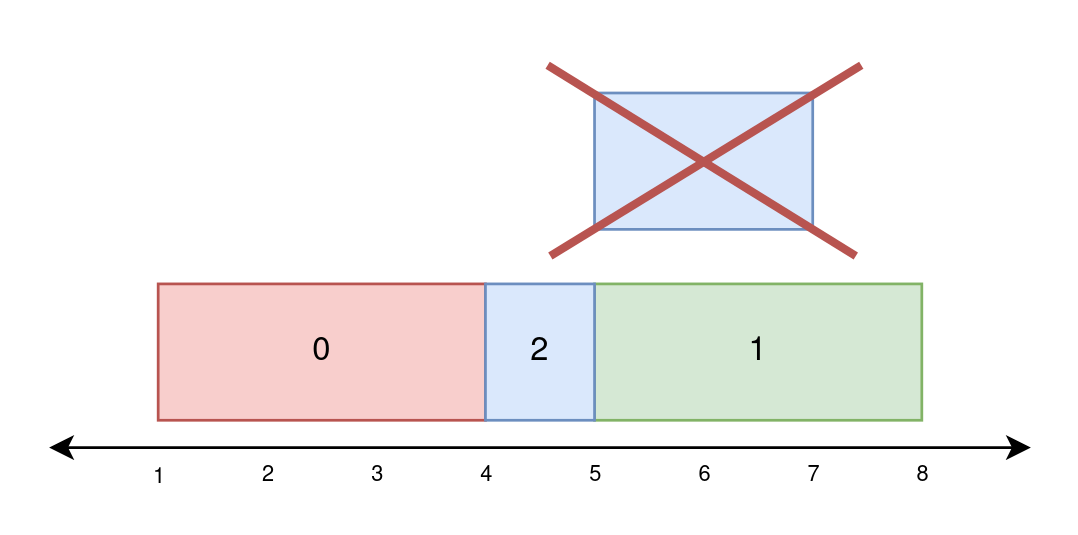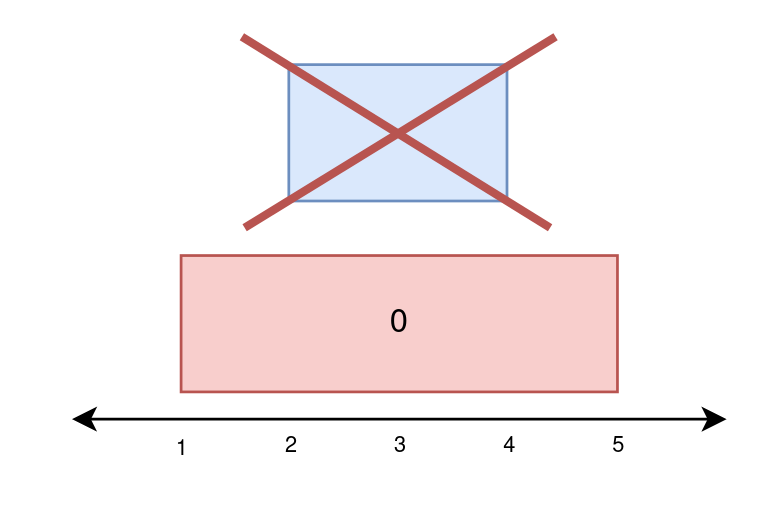# LeetCode 2158. Amount of New Area Painted Each Day Solution

There is a long and thin painting that can be represented by a number line. You are given a 0-indexed 2D integer array{" "} paint of length n, where{" "}  paint[i] = [starti, endi]  . This means that on the{" "}  ith {" "} day you need to paint the area between{" "}  starti {" "} and{" "}  endi  .

Painting the same area multiple times will create an uneven painting so you only want to paint each area of the painting at most once.

Return an integer array worklog of length n , where worklog[i] {" "} is the amount of new area that you painted on the{" "}  ith  day.

Example 1:Input: paint = [[1,4],[4,7],[5,8]]{"\n"}
Output: [3,3,1]{"\n"}
Explanation:
{"\n"}On day 0, paint everything between 1 and 4.{"\n"}The amount of new
area painted on day 0 is 4 - 1 = 3.{"\n"}On day 1, paint everything between
4 and 7.{"\n"}The amount of new area painted on day 1 is 7 - 4 = 3.{"\n"}On
day 2, paint everything between 7 and 8.{"\n"}Everything between 5 and 7 was
already painted on day 1.{"\n"}The amount of new area painted on day 2 is 8
- 7 = 1. {"\n"}


Example 2:Input: paint = [[1,4],[5,8],[4,7]]{"\n"}
Output: [3,3,1]{"\n"}
Explanation:
{"\n"}On day 0, paint everything between 1 and 4.{"\n"}The amount of new
area painted on day 0 is 4 - 1 = 3.{"\n"}On day 1, paint everything between
5 and 8.{"\n"}The amount of new area painted on day 1 is 8 - 5 = 3.{"\n"}On
day 2, paint everything between 4 and 5.{"\n"}Everything between 5 and 7 was
already painted on day 1.{"\n"}The amount of new area painted on day 2 is 5
- 4 = 1. {"\n"}


Example 3:Input: paint = [[1,5],[2,4]]{"\n"}
Output: [4,0]{"\n"}
Explanation:
{"\n"}On day 0, paint everything between 1 and 5.{"\n"}The amount of new
area painted on day 0 is 5 - 1 = 4.{"\n"}On day 1, paint nothing because
everything between 2 and 4 was already painted on day 0.{"\n"}The amount of
new area painted on day 1 is 0.{"\n"}


Constraints:

•  1 <= paint.length <= 105 
• paint[i].length == 2
•  0 <= starti < endi <= 5 * 104 

## Naive solution in $\mathcal{O}(nm)$

Let's split the number line into blocks such that for the $i$th block covers the interval $[i, i+1]$. Create a boolean array to store whether each block has been painted.

On day $i$, we are tasked with painting blocks $\text{start}_i$ to $\text{end}_i-1$. We can check each of these blocks, painting the unpainted ones (we also keep count of how many blocks we paint because that's what the question asks for). In the worst case, we have to check every block on every day. Let $n$ be the number of days (up to $100000$) and let $m$ be the largest number that appears in the input (up to $50000$). The time complexity is $\mathcal{O}(nm)$. This is not fast enough.

## A simple solution in $\mathcal{O}((n+m) \log m)$

Instead of using a boolean array, we can use a BBST (balanced binary search tree) to store the indices of the unpainted blocks. At the start, we insert $0, 1, 2, \ldots, m-1, m$ into the BBST. When we paint a node, we delete its node from the BBST. In our time complexity analysis, it will become clear why we chose to use a BBST.

On each day, we search for the first node $\ge \text{left}_i$. If it's also $< \text{right}_i$, we delete it. We repeatedly do this until there are no more blocks between $\text{left}_i$ and $\text{right}_i-1$.

<mark style={{ backgroundColor: "lightblue" }}>The intuition behind this solution is that we don't want need to needlessly loop over painted blocks; as soon as a block is painted, it's no longer useful, so we delete it. Otherwise, in future days, we'd have to keep checking whether each block has been painted. A BBST can do what we need: find and delete single items quickly.

### Time complexity

Inserting $0, 1, 2, \ldots, m-1, m$ into the BBST at the start takes $\mathcal{O}(m \log m)$ time.

Finding the first node $\ge \text{left}_i$ and deleting a node both take $\mathcal{O}(\log m)$, and we do them at most $n+m$ and $m$ times, respectively.

In total, our algorithm takes $\mathcal{O}(m \log m + (n+m) \log m + m \log m) = \mathcal{O}((n+m) \log m)$.

### Space complexity

A BBST of $m$ elements takes $\mathcal{O}(m)$ space.

### Built-in BBSTs

Most programming languages have built-in BBSTS so we don't have to code them ourselves. C++ has set, Java has TreeSet, Python has SortedList, and JavaScript has SortedSet (but it's not supported on LeetCode).

## C++ Solution

1class Solution {
2public:
3    vector<int> amountPainted(vector<vector<int>>& paint) {
4        set<int> unpainted;
5        vector<int> ans(paint.size());
6        for (int i = 0; i <= 50000; i++) {
7            unpainted.insert(i);
8        }
9        for (int i = 0; i < paint.size(); i++) {
10            int left = paint[i], right = paint[i];
11            // Repeatedly delete the first element >= left until it becomes >= right
12            // This clears values in [left, right) from the set
13            for (auto it = unpainted.lower_bound(left); *it < right; it = unpainted.erase(it), ans[i]++);
14        }
15        return ans;
16    }
17};

## Java Solution

1class Solution {
2    public int[] amountPainted(int[][] paint) {
3        TreeSet<Integer> unpainted = new TreeSet<>();
4        int[] ans = new int[paint.length];
5        for (int i = 0; i <= 50000; i++) {
7        }
8        for (int i = 0; i < paint.length; i++) {
9            int left = paint[i], right = paint[i];
10            // Repeatedly delete the first element >= left until it becomes >= right
11            // This clears values in [left, right) from the TreeSet
12            while (true) {
13                int next = unpainted.ceiling(left);
14                if (next >= right)
15                    break;
16                unpainted.remove(next);
17                ans[i]++;
18            }
19        }
20        return ans;
21    }
22}

## Python Solution

1from sortedcontainers import SortedList
2
3class Solution:
4    def amountPainted(self, paint: List[List[int]]) -> List[int]:
5        unpainted = SortedList([i for i in range(0, 50001)])
6        ans = [0 for _ in range(len(paint))]
7        for i in range(len(paint)):
8            left, right = paint[i]
9            # Repeatedly delete the first element >= left until it becomes >= right
10            # This clears values in [left, right) from the SortedList
11            while unpainted[ind := unpainted.bisect_left(left)] < right:
12                unpainted.__delitem__(ind)
13                ans[i] += 1
14        return ans

## JavaScript Solution

1var SortedSet = require("collections/sorted-set");
2/**
3 * @param {number[][]} paint
4 * @return {number[]}
5 */
6var amountPainted = function (paint) {
7  const n = paint.length;
8  const ans = new Array(n).fill(0);
9  const unpainted = new SortedSet(Array.from(Array(50001).keys()));
10  for (let i = 0; i < n; i++) {
11    (left = paint[i]), (right = paint[i]);
12    // Repeatedly delete the first element >= left until it becomes >= right
13    // This clears values in [left, right) from the SortedSet
14    while ((node = unpainted.findLeastGreaterThanOrEqual(left)).value < right) {
15      unpainted.delete(node.value);
16      ans[i]++;
17    }
18  }
19  return ans;
20};

## Alternative $\mathcal{O}(n \log n)$ solution

Instead of storing the unpainted blocks, we can store the painted segments. We store them as (left, right) pairs in a BBST, where no segments intersect. Each day, we delete segments fully contained in [left_i, right_i], then merge partially overlapping segments with it, all while keeping count of how many blocks we've painted this day. We create, delete, and check for overlaps in $\mathcal{O}(n)$ segments for a total time complexity of $\mathcal{O}(n \log n)$. This solution is trickier to implement—code will not be presented here.